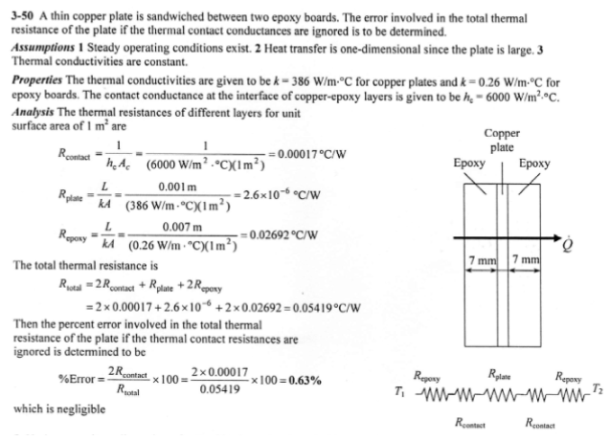heat and mass transfer problems engineering equations heat and mass transfer school homework engineering heat and mass transfer formulas heat and mass transfer solutions to heat and mass transfer problems full solution engineering problem solution heat and mass transfer math problems engineering equations heat and mass transfer school homework engineering solutions to heat and mass transfer formulas heat problems mass problem solutions to transfer problems full solution heat and mass transfer
heat and mass transfer problems engineering equations heat and mass transfer school homework engineering heat and mass transfer formulas heat and mass transfer solutions to heat and mass transfer problems full solution engineering problem solution heat and mass transfer math problems engineering equations heat and mass transfer school homework engineering solutions to heat and mass transfer formulas heat problems mass problem solutions to transfer problems full solution heat and mass transfer
Highalphabet Home Page heat and mass transfer problem solutions Heat and Mass Transfer Page
A 1-mm-thick copper plate (k=386 W/mK) is sandwiched between two 7-mm-thick epozy boards (k=0.26 W/mK) that are 15 cm x 20 cm in size. If the thermal contact conductance on both sides of the copper plate is estimated to be 6000 W/mK, determine the error involved in the total thermal resistance of the plate if the thermal contact conductances are ignored.A 1-mm-thick copper plate (k=386 W/mK) is sandwiched between two 7-mm-thick epozy boards (k=0.26 W/mK) that are 15 cm x 20 cm in size. If the thermal contact conductance on both sides of the copper plate is estimated to be 6000 W/mK, determine the error involved in the total thermal resistance of the plate if the thermal contact conductances are ignored.